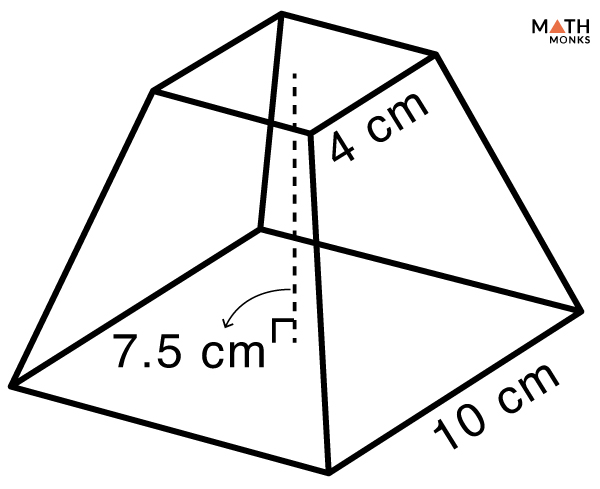# Volume of a Pyramid

The volume of a pyramid is the space it occupies in a 3-dimensional plane. It is expressed in cubic units such as m3, cm3, mm3, and in3.

## Formulas

The general formula to find the volume of any pyramid is:

Volume (V) = ${\dfrac{1}{3}Bh}$, here B = base area, h = height

However, there are specific formulas to calculate the volume of different pyramids due to their different shapes. They are:

Let us solve some examples to understand the above concept better.

## Solved Examples

Find the volume of a triangular pyramid with a base area of 64 cm2 and a height of 6.3 cm.

Solution:

As we know,
Volume (V) = ${\dfrac{1}{3}Bh}$, here B = 64 cm2, h = 6.3 cm
∴ V = ${\dfrac{1}{3}\times 64\times 6.3}$
= 134.4 cm3

Finding the volume of a triangular pyramid when the BASE LENGTH, BASE HEIGHT and HEIGHT are known

Find the volume of a triangular pyramid whose height is 6 cm, base length is 7 cm, and base height is 8.5 cm.

Solution:

As we know,
Volume (V) = ${\dfrac{1}{6}bHh}$ , here b = 7 cm, H = 6 cm, h = 8.5 cm
∴ V = ${\dfrac{1}{6}\times 7\times 6\times 8.5}$
= 59.5 cm3

Finding the volume of an equilateral triangular pyramid when the BASE LENGTH and HEIGHT are known

Find the volume of a triangular pyramid whose height is 18 cm, and base is 4 cm each.

Solution:

As we know,
The area of an equilateral triangle = ${\dfrac{\sqrt{3}}{4}b^{2}}$, here a = side of the triangle
Using this formula,
Volume (V) = ${\dfrac{\sqrt{3}}{12}b^{2}h}$ , here b = 4 cm, h = 18 cm
∴ V = ${\dfrac{\sqrt{3}}{12}\times 4^{2}\times 18}$
= 18.47 cm3

Find the volume of a square pyramid whose height is 13 cm, and base is 5 cm.

Solution:

As we know,
Volume (V) = ${\dfrac{1}{3}b^{2}h}$, here b = 5 cm, h = 13 cm
∴ V = ${\dfrac{1}{3}\times 5^{2}\times 13}$
= 108.33 cm3

Find the volume of a rectangular pyramid whose height is 8 cm, and bases are 6 cm and 9 cm.

Solution:

As we know,
Volume (V) = ${\dfrac{1}{3}lwh}$, here l = 9 cm, w = 6 cm, h = 8 cm
∴ V = ${\dfrac{1}{3}\times 9\times 6\times 8}$
= 144 cm3

Find the volume of a hexagonal pyramid whose height is 7 cm, and base is 3 cm.

Solution:

As we know,
Volume (V) = ${\dfrac{\sqrt{3}}{2}b^{2}h}$ , here b = 3 cm, h = 7 cm
∴ V = ${\dfrac{\sqrt{3}}{2}\times 3^{2}\times 7}$
= 554.55 cm3

Finding the volume of a hexagonal pyramid when the BASE, APOTHEM, and HEIGHT are known

Find the volume of a hexagonal pyramid with a height of 14 cm, base of 5 cm, and apothem of 4.3 cm.

Solution:

Here we will use an alternative formula to calculate the volume.
Volume (V) = abh, here a = 4.3 cm, b = 5 cm, h = 14 cm
∴ V = 4.3 × 5 × 14
= 301 cm3

Find the volume of a pentagonal pyramid with a height of 11 cm, base of 7 cm, and apothem of 4.81 cm.

Solution:

As we know,
Volume (V) = ${\dfrac{5}{6}abh}$, here a = 4.81 cm, b = 7 cm, h = 11 cm
∴ V = ${\dfrac{5}{6}\times 4.81\times 7\times 11}$
= 308.64 cm3

Finding the volume of a pentagonal pyramid when the BASE and HEIGHT are known

Find the volume of a pentagonal pyramid with a height of 9.5 cm, and base of 6 cm.

Solution:

Here we will use an alternative formula to calculate the volume.
Volume (V) = ${\dfrac{1.72}{3}b^{2}h}$, here b = 6 cm, h = 9.5 cm
∴ V = ${\dfrac{1.72}{3}\times 6^{2}\times 9.5}$
= 196.08 cm3Find the volume of a given truncated pyramid.

Solution:

Volume (V) = ${\dfrac{1}{3}h^{2}\left( a^{2}+b^{2}+ab\right)}$, here a = 10 cm, b = 4 cm, h = 7.5 cm
∴ V = ${\dfrac{1}{3}\times 7.5^{2}\times \left( 10^{2}+4^{2}+10\times 4\right)}$
= 390 cm3